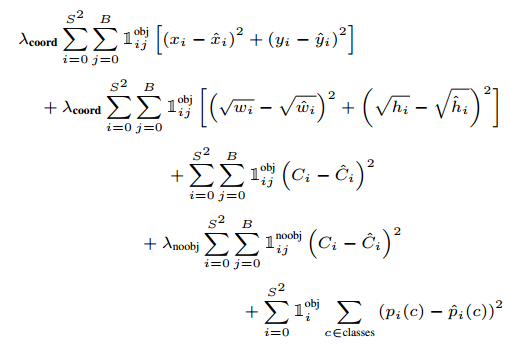## Summary

YOLO is a unified solution to the object detection problem. Other approches like R-CNN are using a two step process (localization and classification) where YOLO is a one step process. Also, Fast YOLO (or tiny-yolo) is achieving 155 fps where Faster R-CNN is doing 18 fps.

On the Pascal VOC-2012 dataset, YOLO is achieving 63.4 mAP where Faster R-CNN is doing 73.2. In this case, Faster R-CNN is not real-time (7 fps) while YOLO is doing around 60 fps.

Model Mean avg precision FPS
Faster R-CNN 73.2 7
YOLO 63.4 45
Fast-YOLO 52.7 155

It’s important to know that YOLO V1 only supports a single aspect ratio, this is fixed in YOLOV2 with the use of prior boxes and this is the main drawback if we compare YOLOV1 to Single shot detector (SSD). Because we are not using prior boxes, YOLO has no starting point which makes it hard to train.

## Output

Since YOLO is predicting multiple boxes per cell, the output is a dense layer with {nb_detection * (4 + nb_classes + 1)} dimensions. For each cell, the network predicts the boundaries (x,y,w,h), a probability vector of the classes and the probability that there is an object in this cell. x,y are relative to the top-left corner of the cell and the w,h are computed as the proportion of the entire frame.

## Loss

The loss function is quite simple although there is a lot of parameters that you need to tune.The values of the predicted boxes are penalized with a MSE if there is an object in this cell.

It is important to know that because YOLO predicts multiple boxes per cell, only the predicted box with the biggest intersection over union (IOU) with the ground truth is used to compute the loss.

## Implementations

Darknet (Original C++ code)

Darkflow (Tensorflow)

Darknet.keras (Keras + Tensorflow)